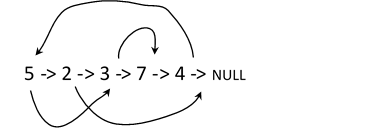# Copy list with random Pointer in C++

C++Server Side ProgrammingProgramming

A Linked List is a linear data structure in which each node is having two blocks such that one block contains the value or data of the node and the other block contains the address of the next field.

Let us assume that we have a linked list such that each node contains a random pointer which is pointing to other nodes in the list. The task is to construct the list with the same as the original list. Copying the list from the original list which is having some random pointer is called a 'Deep Copy' of the linked list.

For Example

Input-1Output:

5-> 2 -> 3 -> 7 ->4 ->

Explanation: If we append the new list with the value of the original nodes in the given linked list and replace the random pointer of the original linked list with the next node in the new list, then it will become 5-> 2- >3 -> 7-> 4->

## Approach to Solve this Problem

We have a linked list with nodes containing its data and a random pointer. To achieve the copy of the linked list with the data and random pointer, we will first append the new node with the same value after each node. This will create a duplicate node after each node.

After initialization, check the path of the random pointer in the list and place the random pointer accordingly into the newly created node.

Now separating the newly created nodes after each node in the original list will create a deep copy of the Linked List.

• Take a linked list with the data field and pointer to the address of its random node.
• A function copyRandomList(listnode*head) takes the head node of the original list as the input and returns the deep copy of the list.
• If the head is empty, then the list is empty and we have to return the head as well.
• Now insert a new node with the same value after each node of the original list.
• Then copy the random pointer from the original list and insert the new nodes, i.e., newnode->next = curr->randomPointer
• Once a new node is created with the pointer and data, we will separate the list and return the list as the output.

## Example

Live Demo

#include <bits/stdc++.h>
using namespace std;
struct listnode {
int data;
listnode * next, * random;
listnode(int d) {
data = d;
next = NULL;
random = NULL;
}
};
while (curr) {
cout << curr -> data << " " << curr -> random -> data << endl;
curr = curr -> next;
}
}
listnode * copyRandomList(listnode * head) {
}
//Insert a new node with the same value after each node in the original list.
while (curr) {
listnode * newHead = new listnode(curr -> data);
newHead -> next = curr -> next;
curr = curr -> next -> next;
}
//Now place the randompointer with the newly created node.
while (curr) {
if (curr -> random)
(curr -> next) -> random = (curr -> random) -> next;
curr = curr -> next -> next;
}
//Now Let us separate the newly created list
listnode * result = curr -> next;
listnode * dummyHead = new listnode(-1);
while (curr) {
curr -> next = result -> next;
curr = curr -> next;

if (curr) {
result -> next = curr -> next;
}
result = result -> next;
}
return result;
}
int main() {
listnode * head = new listnode(5);
head -> next = new listnode(6);
head -> next -> next = new listnode(3);
head -> next -> next -> next = new listnode(4);
head -> next -> next -> next -> next = new listnode(2);
head -> next -> next -> random =
head -> next -> next -> next -> next;
head -> next -> next -> next -> random =
head -> next -> next -> next -> next;
head -> next -> next -> next -> next -> random =
cout << "Original list :" << endl;
cout << "Deep Copy of the List:" << endl;
print(deep_copyList);
return 0;
}

Running the above code will generate the output as,

## Output

Original List:
5 3
6 5
3 2
4 2
2 6
Deep Copy of the List:
5 3
6 5
3 2
4 2
2 6